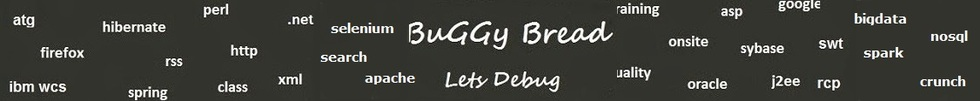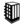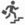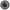What is recursion in Java ?# Search Interview QuestionsMore than 3000 questions in repository.There are more than 900 unanswered questions.Have a video suggestion.
Click Correct / Improve and please let us know.
Label / Company      Label / Company / TextSubmit Question

Interview Questions and AnswersQ1. What is recursion in Java ? Core Java
Ans. It's a programming technique wherein the method can call itself. The method call usually involves a different set of parameters as otherwise it would lead to an infinite loop. There is usually a termination check and statement to finally return the control out of all function calls.Help us improve. Please let us know the company, where you were asked this question :LikeDiscussCorrect / ImproveAsked in 1 CompaniesBasicRelated QuestionsWhat will happen if we don't have termination statement in recursion ?Write a Program to print factorial of a number using recursionFind Fibonacci first n numbers using recursion ?Write an Algorithm for Graph Traversal ? The Graph has a loop.Write a program to print sum of numbers between the start and end number

For example - Passing start number as 2 and end number as 10, it should print 2+3+4+5+6+7+8+9+10 = 54Write a program using Recursion to print multiplication of numbers between the start and end number

For example - Passing start number as 2 and end number as 10, it should print 2*3*4*5*6*7*8*9*10 = 3628800What will happen if we don't have termination statement in recursion ?Which of the two - iteration or recursion - is slower ?Write a Program to print fibonacci series using recursion.

## Help us and Others Improve. Please let us know the questions asked in any of your previous interview.

Any input from you will be highly appreciated and It will unlock the application for 10 more requests.

 Company Name: Questions Asked: